# 陆水水库洪水集合概率预报方法与应用研究Ensemble Probability Flood Forecasting Method and Application in Lushui Reservoir

DOI: 10.12677/JWRR.2020.96061, PDF, 下载: 124  浏览: 223  国家自然科学基金支持

Abstract: To explore the uncertainty of hydrologic model structure in the leading time flood forecasting accuracy, we selected the dataset of Lushui Reservoir watershed and the precipitation forecast data of the European Center for Medium-Term Weather Forecast (ECMWF) to drive the three hydrological models (API, Xinanjiang and GR4J). Through the analysis of the expected value forecast accuracy and the overall per-formance of the probability forecast, the effectiveness of the two statistical post-processing methods, the Bayesian model averaging (BMA) and the improved BMA (M-BMA) were compared. The results show that the expected value forecast of the two methods improves the original forecast accuracy to a certain extent; both methods can provide reliable forecast intervals; through the analysis of multiple indicators such as Continuous ranked probability score (CRPS), the performance of the M-BMA method is better than that of the BMA method.

1. 引言

2. 研究方法

2.1. 贝叶斯模型加权平均方法

$p\left(h|{s}_{1},{s}_{2},\cdots ,{s}_{i},H\right)=\underset{i=1}{\overset{k}{\sum }}p\left({s}_{i}|H\right)\cdot p\left(h|{s}_{i},H\right)$ (1)

$\stackrel{^}{s}=\left\{\begin{array}{l}\frac{{s}^{\theta }-1}{\theta }\text{\hspace{0.17em}}\text{\hspace{0.17em}}\text{\hspace{0.17em}}\text{\hspace{0.17em}}\text{\hspace{0.17em}}\text{\hspace{0.17em}}\text{\hspace{0.17em}}\theta \ne 0\\ \mathrm{ln}\left(s\right)\text{\hspace{0.17em}}\text{\hspace{0.17em}}\text{\hspace{0.17em}}\text{\hspace{0.17em}}\text{\hspace{0.17em}}\text{\hspace{0.17em}}\text{\hspace{0.17em}}\text{ }\theta =0\end{array}$ (2)

Sloughter  等对BMA方法进行了改进，假设非正态分布变量服从Gamma分布，即公式(1)中的 $p\left(h|{s}_{i},H\right)$ 可由Gamma分布概率密度函数来表达：

$h\left(x|\alpha ,\beta \right)=\frac{1}{{\beta }^{\alpha }\cdot \Gamma \left(\alpha \right)}\cdot {x}^{\alpha -1}\cdot {\text{e}}^{\frac{-x}{b}},x>0$ (3)

$\alpha =\frac{{\mu }^{2}}{{\sigma }^{2}},\text{\hspace{0.17em}}\text{\hspace{0.17em}}\beta =\frac{{\sigma }^{2}}{\mu }$ (4)

$\begin{array}{c}\mu ={b}_{0k}+{b}_{1k}\cdot {s}_{k},\text{\hspace{0.17em}}\text{\hspace{0.17em}}{\sigma }^{2}={\left({c}_{0k}+{c}_{1k}\cdot {s}_{k}\right)}^{2}\end{array}$ (5)

M-BMA方法的表达式为：

$p\left(h|{s}_{1},{s}_{2},\cdots ,{s}_{k}\right)=\underset{i=1}{\overset{k}{\sum }}{w}_{i}\cdot {g}_{i}\left(h|{s}_{i}\right)$ (6)

$E\left(h|{s}_{1},{s}_{2},\cdots ,{s}_{k}\right)={\int }_{0}^{+\infty }h\cdot p\left(h|{s}_{1},{s}_{2},\cdots ,{s}_{i}\right)\text{d}h$ (7)

2.2. 模型评价指标

2.2.1. 确定性预报

1) 纳什效率系数(NSE)

$\text{NSE}=\left(1-\frac{{\sum }_{t=1}^{T}{\left({h}_{t}-{s}_{t}\right)}^{2}}{{\sum }_{t=1}^{T}{\left({h}_{t}-\stackrel{¯}{h}\right)}^{2}}\right)×100%$ (8)

2) 径流总量相对误差(RE)

$\text{RE}=\frac{{\sum }_{t=1}^{T}{s}_{t}-{\sum }_{t=1}^{T}{h}_{t}}{{\sum }_{t=1}^{T}{h}_{t}}×100%$ (9)

3) 平均绝对误差(MAE)

$\text{MAE}=\frac{{\sum }_{t=1}^{T}|{h}_{t}-{s}_{t}|}{T}$ (10)

NSE表明预报流量与实测流量模拟精度，NSE越接近于1，表明精度越高，模型方法越优。RE的绝对值越小，表明实测流量总量和预报流量总量误差越小。MAE表征预报流量与实测流量之间的平均误差，MAE越小，精度越高。

2.2.2. 概率预报

1) Q-Q图。Q-Q图通过比较预报值概率分布与实测值概率分布的差异，从概率角度检验预报概率分布的准确性 。设第t个时段的预报累积分布函数为 ${F}_{t}\left(x\right)$ ，P值是第t个时段实测流量 ${h}_{t}$ 对应的预报累积概率分布函数值，记为 ${P}_{t}={F}_{t}\left({h}_{t}\right)$ 。如果预报概率分布与实测值的概率分布相同，则 ${P}_{t}$ 符合标准均匀分布 $U~\left(0,\text{1}\right)$ ，对应于Q-Q图中的1:1线，否则就反映了预报概率的偏差。Q-Q图曲线越接近于1:1线，概率预报结果越合理。图1为概率预报Q-Q示意图 。

2) 可靠性(α_index)。α_index用来定量描述可靠性的高低 ，计算公式如下：

$\begin{array}{c}\alpha \text{_index}=1-\frac{2}{T}\underset{t=1}{\overset{T}{\sum }}\left(|{q}^{em}\left({P}_{t}\right)-{q}^{th}\left({P}_{t}\right)|\right)\end{array}$ (11)

3) 连续概率排位分数(CRPS)。连续概率排位分数是结合可靠性和集中度的综合指标，是评估概率预报结果总体效果的标准方法 ，数学表达式为：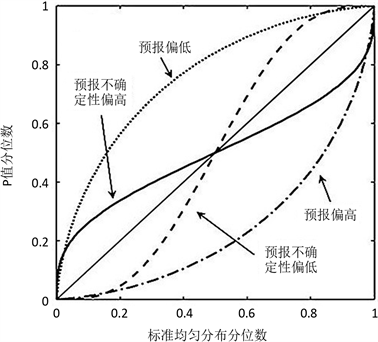Figure 1. Schematic diagram of probability forecast Q-Q

$\text{CRPS}=\frac{1}{T}\underset{t=1}{\overset{T}{\sum }}{\int }_{0}^{+\infty }{\left({F}_{t}\left(r\right)-{H}_{s}\left(r-{h}_{t}\right)\right)}^{2}\text{d}r$ (12)

4) 覆盖率(CR)和平均带宽(B)。覆盖率是指预报区间覆盖实测流量数据的比率，是最常用的预报区间评价指标。

$\text{CR}=\frac{n}{T}$ (13)

$\text{B}=\frac{1}{T}\underset{t=1}{\overset{T}{\sum }}\left({h}_{tu}-{h}_{tl}\right)$ (14)

5) 单位平均相对区间宽度所包含的实测点据比例(PUCI)

$\text{PUCI}=\frac{\text{CR}}{\text{RB}}$ (15)

3. 水文模拟预报结果

3.1. 研究流域及数据

3.2. 水文模拟结果分析Table 1. Calibration and verification results of Xinanjiang and GR4J models

3.3. 考虑降水预报的洪水预报结果分析

API模型由降雨径流相关图和单位线构成，属于多输入、单输出静态的系统数学模型 。API模型是陆水水库实际作业预报采用的主要预报模型，使用效果良好。本文基于API、新安江、GR4J三个模型，以流域实测的面平均降水和欧洲中心数值天气预报数据作为输入，以2014~2019年汛期(5月1日~11月1日)每日8:00为基准点，计算得到预见期1~4个时段(时段为3 h)陆水水库入库流量的预报结果。表2列出1~4个时段预见期的API、新安江和GR4J模型预报入库流量的各项评价指标。Table 2. Evaluation of flood forecasting results in Lushui Reservoir

4. 集合概率预报结果评价

4.1. 自适应滑动窗口

4.2. 集合概率预报期望值评价

Table 3. Comparison of deterministic forecast results in different lead times

4.3. 概率预报结果评价Table 4. Evaluation index value of 90% forecast interval for different leading times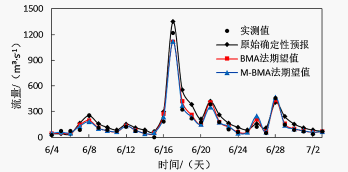(a) 预见期3 h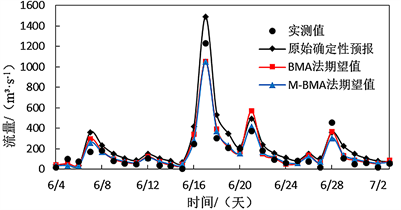(a) 预见期6 h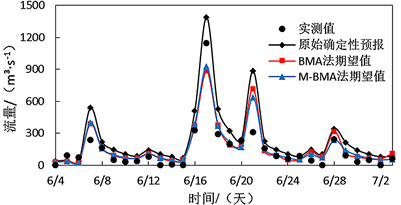(c) 预见期9 h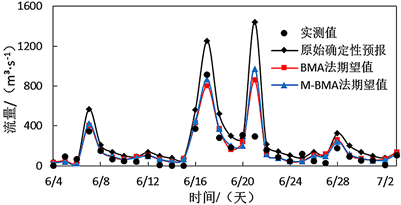(d) 预见期12 h

Figure 2. The deterministic forecast and the expected value of the two methods for different lead times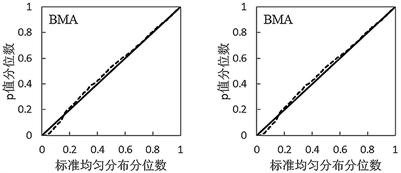(a) 预见期3 h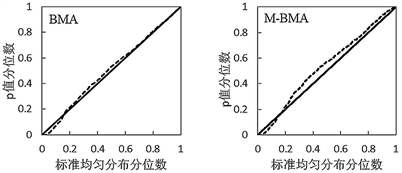(a) 预见期6 h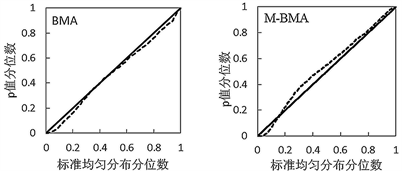(c) 预见期9 h(d) 预见期12 h

Figure 3. Flow probability forecast Q-Q diagrams of BMA and M-BMA methods in different lead times

5. 结论

1) 三种模型方案预报中，GR4J模型和新安江模型预报结果整体表现相当，API模型预报结果整体表现较差，但GR4J模型方案水量误差相对较小，新安江模型次之，API模型方案水量误差最大。

2) 纳什效率系数(NSE)等指标表明，经统计后处理方法校正过的预报值在一定程度上提高了预报精度。两种后处理方法得到的期望值预报效果差距较小，均与实测流量序列拟合效果较好，且优于确定性预报结果，随着预见期的延长，概率预报期望值的精度会相应降低。

3) BMA和M-BMA方法不同预见期覆盖率CR值接近指定置信水平90%，因此90%预报区间是可靠的，由PUCI指标可知，M-BMA方法的预报区间表现更为优良，可以为防洪决策提供更多的预报风险信息。随着预见期的延长，平均带宽逐渐增大，入库流量的不确定性增加。

4) BMA和M-BMA方法得出的概率预报分布均能较为可靠地模拟出实测流量概率分布的统计特征。由CRPS指标可知，经统计后处理的预报值的整体表现要优于确定性预报值，且可靠性较高；M-BMA方法相较于BMA方法整体表现更为优良。随着预见期的延长，两种方法的概率预报性能和总体效果降低。总体而言，M-BMA方法应用灵活，不需要正态变换，预报效果良好，有良好的研究前景。

  张建云. 中国水文预报技术发展的回顾与思考[J]. 水科学进展, 2010, 21(4): 435-443. ZHANG Jianyun. Review and reflection on China’s hydrological forecasting techniques. Advances in Water Science, 2010, 21(4): 435-443. (in Chinese)  陆桂华, 吴娟, 吴志勇. 水文集合预报试验及其研究进展[J]. 水科学进展, 2012, 23(5): 728-734. LU Guihua, WU Juan and WU Zhiyong. Advances in hydrologic ensemble prediction experiment. Advances in Water Science, 2012, 23(5): 728-734. (in Chinese)  刘章君, 郭生练, 李天元, 等. 贝叶斯概率洪水预报模型及其比较应用研究[J]. 水利学报, 2014, 45(9): 1019-1028. LIU Zhangjun, GUO Shenglian, LI Tianyuan, et al. Comparative study of Bayesian probabilistic flood forecasting models. Journal of Hydraulic Engineering, 2014, 45(9): 1019-1028. (in Chinese)  彭勇, 徐炜, 王萍, 等. 耦合TIGGE降水集合预报的洪水预报[J]. 天津大学学报(自然科学与工程技术版), 2015, 48(2): 177-184. PENG Yong, XV Wei, WANG Ping, et al. Flood forecasting coupled with TIGGE ensemble precipitation forecasts. Journal of Tianjin University (Science and Technology), 2015, 48(2): 177-184. (in Chinese)  LIU, Y. Q., GUPTA, H V. Uncertainty in hydrologic modeling: Toward an integrated data assimilation framework. Water Resources Research, 2007, 43(7): 1-18. https://doi.org/10.1029/2006WR005756  MARTY, R., FORTIN, V., KUSWANTO, H., et al. Combining the Bayesian processor of output with Bayesian model averaging for reliable ensemble forecasting. Journal of the Royal Statistical Society: Series C (Appl. Stat.), 2015, 64(1): 75-92. https://doi.org/10.1111/rssc.12062  DAI, K., ZHU, Y. J. and BI, B. G. The review of statistical post-process technol-ogies for quantitative precipitation forecast of ensemble prediction system. Acta Meteorologica Sinica, 2018, 76(4): 493-510.  RAFTERY, A. E., BALABDAOUI, F. and Gneiting, T. Using Bayesian model averaging to calibrate forecast ensembles. Monthly Weather Review, 2005, 133(5): 1155-1174. https://doi.org/10.1175/MWR2906.1  董磊华, 熊立华, 万民. 基于贝叶斯模型加权平均方法的水文模型不确定性分析[J]. 水利学报, 2011, 42(9): 1065-1074. DONG Leihua, XIONG Lihua and WAN Min. Uncertainty analysis of hydrological modeling using the Bayesian model aver-aging method. Journal of Hydraulic Engineering, 2011, 42(9): 1065-1074. (in Chinese)  刘章君, 郭生练, 许新发, 等. 贝叶斯概率水文预报研究进展与展望[J]. 水利学报, 2019, 50(12): 1467-1478. LIU Zhangjun, GUO Shenglian, XV Xinfa, et al. Bayesian probabilistic hydrological forecasting: Progress and prospects. Journal of Hydraulic Engineering, 2019, 50(12): 1467-1478. (in Chinese)  SLOUGHTER, J. M., GNEITING, T. and RAFTERY, A. E. Probabilistic wind speed forecasting using ensembles and Bayesian model averaging. Journal of the American Statistical Association, 2010, 105(489): 25-35. https://doi.org/10.1198/jasa.2009.ap08615  巴欢欢, 郭生练, 钟逸轩, 等. 考虑降水预报的三峡入库洪水集合概率预报方法比较[J]. 水科学进展, 2019, 30(2): 186-197. BA Huanhuan, GUO Shenglian, ZHONG Yixuan, et al. Comparative study on probabilistic ensemble flood forecasting consi-dering precipitation forecasts for the Three Gorges Reservoir. Advances in Water Science, 2019, 30(2): 186-197. (in Chi-nese)  DARBANDSARI, P., COULIBALY, P. Inter-Comparison of different Bayesian model averaging modifications in streamflow simulation. Water, 2019, 11(8): 1707. https://doi.org/10.3390/w11081707  THYER, M., RENARD, B. J., KAVETSKI, D., et al. Critical evaluation of parameter consistency and predictive uncertainty in hydrological modeling: A case study using Bayesian total error analysis. Water Resources Research, 2009, 45(12): 1-22. https://doi.org/10.1029/2008WR006825  李明亮, 杨大文, 陈劲松. 基于采样贝叶斯方法的洪水概率预报研究[J]. 水力发电学报, 2011, 30(3): 27-33. LI Mingliang, YANG Dawen and CHEN Jinsong. Probabilistic flood forecasting by a sampling-based Bayesian model. Journal of Hydroelectric Engineering, 2011, 30(3): 27-33. (in Chinese)  ENGELAND, K., STEINSLAND, I. Probabilistic post processing models for flow forecasts for a system of catchments and several lead times. Water Resources Research, 2014, 50(1): 182-197. https://doi.org/10.1002/2012WR012757  HE, S. K., GUO, S. L., LIU, Z. J., et al. Uncertainty analysis of hydrological multi-model ensembles based on CBP-BMA method. Hydrology Research, 2018, 49(5): 1636-1651. https://doi.org/10.2166/nh.2018.160  邓鹏鑫, 王银堂, 胡庆芳, 等. GR4J模型在赣江流域日径流模拟中的应用[J]. 水文, 2014, 34(2): 60-65. DENG Pengxin, WANG Yintang, HU Qingfang, et al. Application of GR4J in daily runoff simulation for Ganjiang river basin. Journal of China Hydrology, 2014, 34(2): 60-65. (in Chinese)  钟小燕, 文磊, 余钟波. 连续API模型在沂河临沂站径流预报中的应用[J]. 人民长江, 2017, 48(13): 26-30. ZHONG Xiaoyan, WEN Lei and YU Zhongbo. Application of continuous API hydrological model at Linyi Hydrological station of Yihe River. Yangtze River, 2017, 48(13): 26-30. (in Chinese)  ZHONG, Y. X., GUO, S. L., XIONG, F., et al. Probabilistic forecasting based on ensemble forecasts and EMOS method for TGR inflow. Frontiers of Earth Science, 2020, 14(1): 188-200. https://doi.org/10.1007/s11707-019-0773-9  MA, Z. Q., XU, J. T., HE, K., et al. An updated moving window algorithm for hourly-scale satellite precipitation downscaling: A case study in the Southeast Coast of China. Journal of Hydrology, 2019, 581: 1-42. https://doi.org/10.1016/j.jhydrol.2019.124378